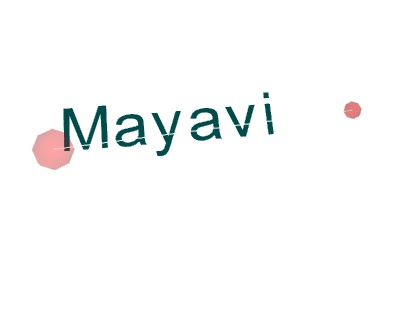# Tvtk in mayavi example¶

An example of pure TVTK programming to build TVTK objects, which are then added to a Mayavi scene.

This example show how pure TVTK objects can be added to a Mayavi scene.

This programming style does not allow to benefit from the data-management facilities of Mayavi (the pipeline, the data-oriented mlab functions), but it allows to easily reuse VTK code together with Mayavi or mlab code.

If you want to use arbritrary VTK filters with Mayavi, it is best to use the UserDefined Mayavi filter, which enables the user to insert any VTK filter in the Mayavi pipeline. See, for instance, the Mri example for example of the UserDefined filter. For a full-blown example of a complex VTK pipeline built with Mayavi, see Tvtk segmentation example.Python source code: `tvtk_in_mayavi.py`

```
# Author: Gael Varoquaux <gael.varoquaux@normalesup.org>
# Copyright (c) 2008, Enthought, Inc.

from mayavi import mlab

# To access any VTK object, we use 'tvtk', which is a Python wrapping of
# VTK replacing C++ setters and getters by Python properties and
# converting numpy arrays to VTK arrays when setting data.
from tvtk.api import tvtk
from tvtk.common import configure_input_data

v = mlab.figure()

# Create a first sphere
# The source generates data points
sphere = tvtk.SphereSource(center=(0, 0, 0), radius=0.5)
# The mapper converts them into position in, 3D with optionally color (if
# scalar information is available).
sphere_mapper = tvtk.PolyDataMapper()
configure_input_data(sphere_mapper, sphere.output)
sphere.update()

# The Property will give the parameters of the material.
p = tvtk.Property(opacity=0.2, color=(1, 0, 0))
# The actor is the actually object in the scene.
sphere_actor = tvtk.Actor(mapper=sphere_mapper, property=p)

# Create a second sphere
sphere2 = tvtk.SphereSource(center=(7, 0, 1), radius=0.2)
sphere_mapper2 = tvtk.PolyDataMapper()
configure_input_data(sphere_mapper2, sphere2.output)
sphere2.update()
p = tvtk.Property(opacity=0.3, color=(1, 0, 0))
sphere_actor2 = tvtk.Actor(mapper=sphere_mapper2, property=p)

# Create a line between the two spheres
line = tvtk.LineSource(point1=(0, 0, 0), point2=(7, 0, 1))
line_mapper = tvtk.PolyDataMapper()
configure_input_data(line_mapper, line.output)
line.update()
line_actor = tvtk.Actor(mapper=line_mapper)

# And display text
vtext = tvtk.VectorText()
vtext.text = 'Mayavi'
text_mapper = tvtk.PolyDataMapper()
configure_input_data(text_mapper, vtext.get_output())
vtext.update()
p2 = tvtk.Property(color=(0, 0.3, 0.3))
text_actor = tvtk.Follower(mapper=text_mapper, property=p2)
text_actor.position = (0, 0, 0)